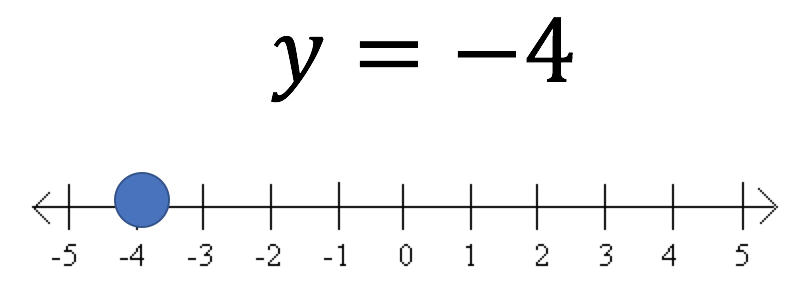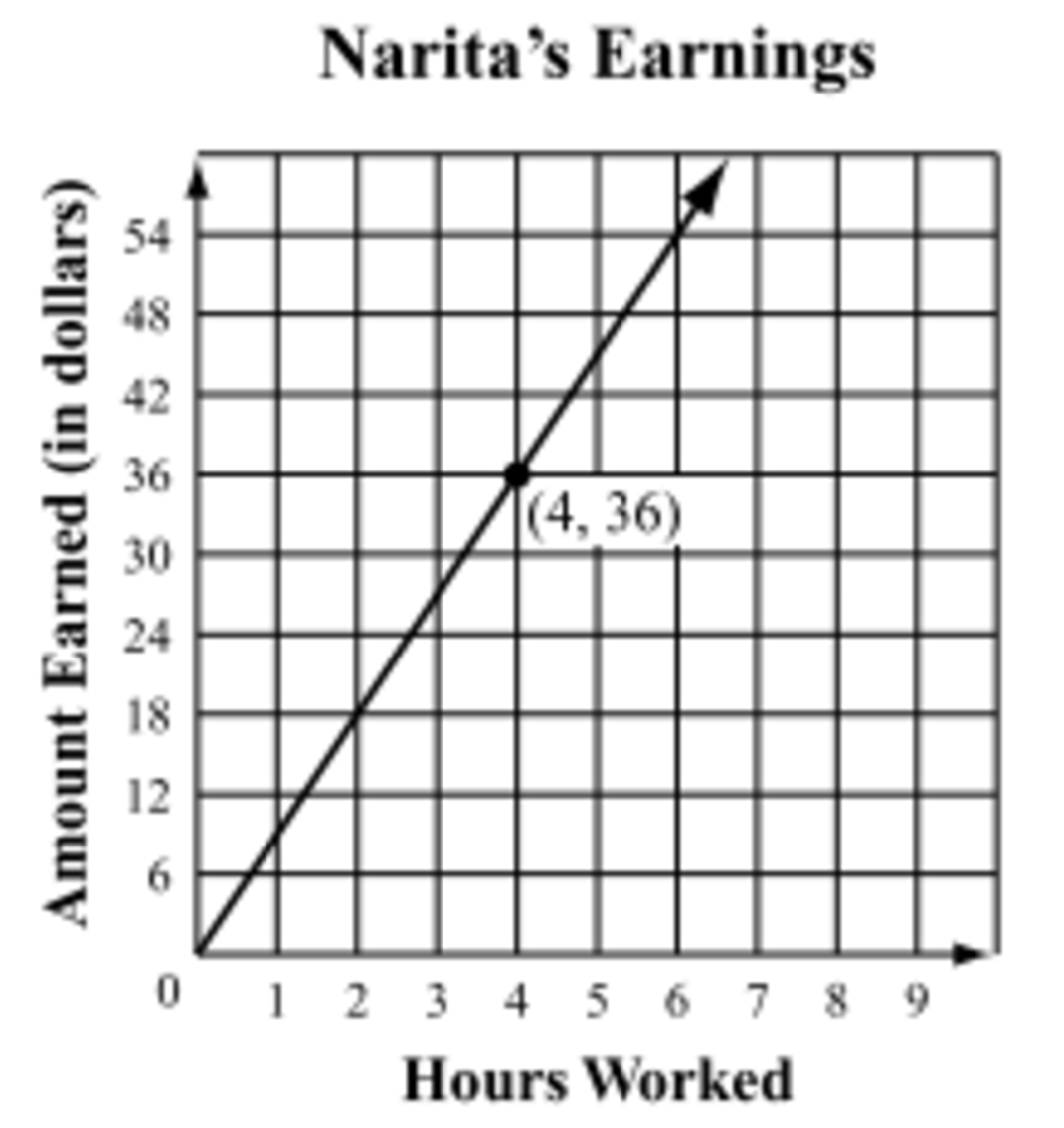The Coordinate Plane
starstarstarstarstarstarstarstarstarstar
by Felix Gabathuler
| 14 Questions
Your mission today is to slowly read through this online lesson and complete all the tasks in the order that they appear. You will watch short videos, complete Khan Academy excercises and worksheets, and share you opinions on certain things. The goal for today is teach yourself the concept of plotting points on the coordinate plane.Today you are going to dive into the magical, mysterious world of the Coordinate Plane! Long ago you learned how to plot numbers on a number line. If not, you pretty much just find the number you're looking for on the number line and draw a dot over it... like this:Now that we know how to solve one-step, two-step, and even three or more step equations involving one variable, we could easily plot that answer on a number line, like you see below.Once we became masters at solving equations, we introduced the question, "What if there are two variables, instead of just one?" Like, what if our equation is y + 3 = x.
1
1
Can I solve for x without knowing what y is?
Yes
No
Equations involving two variables represent hypothetical situations, meaning we can use them to predict or plan for things that have not happened yet. Take the equation y = 10x. We could say that x represents how many hours you babysit per week and 10 represents the amount of money in dollars you earn per hour.
2
1
In this case, the variable y stands for the amount of money you earn each week.
True
False

3
1
How much money would you make in one week if you babysat for 5.5 hours?
\$15.50
\$55.50
\$55.00
155.00
4
1
One week you made a total of \$140, how many hours did you babysit?
140 hours
14 hours
1.4 hours
10 hours
What if we wanted to make a visual representation of how much money you could make given an unkown amount of hours you might work. People do it all the time.5
1
About how much money is Narita making per hour?
\$9
\$8
\$10
\$11
6
1
Can you explain why there is only one correct answer to #5 even though it seems as though it could be any or them?
7
1
Challenge question!! Can you write an equation involving two variables to represent Narita's model? Use the variables x and y.
Well, in order to create models like Narita's above, you have to first understand the concept of the coordinate plane. Below you'll find some videos and excercises to help us become pros at it! Good luck and remember, don't skip anything!
8
1
What's your biggest takeaway from the video you just watched?
Now that you know how to plot points, try your hand with the following Khan Academy Lesson. Click on the link below to access the lesson:

9
1
I received a 100% on the Graph Points lesson on Khan Academy... because if I didn't, I'd have to do it again...
True
False
Let's kick it up a notch with some real world application problems. Watch the video below to get a good idea of how to tackle these scenarios.

and then

10
1
I received a 100% on the Coordinate Plane Parts Review and the Coordinate Planbe Word Problems (Quadrant 1) lessons on Khan Academy... because if I didn't, I'd have to do them again...
True
False
Time to zoom out and take a look at the entire coordinate plane. Try this worksheet. If you need help, watch the attached videos. Good luck.

So what's the point of a coordinate plane? Well, sometimes you can use it ro represent data visually. Since there is an x and y axis, we can assume those stand for two variables in an equation. You can then plug in different numbers into the equation to get various results. Watch the video below about making a table of results given a linear equation.
11
1
What is you biggest takeaway from the video above?
Try the lesson below to test your knowledge.

12
1
I received a 100% on the Solutions to 2-variable equations lesson on Khan Academy... because if I didn't, I'd have to do it again...
True
False
Now combine the two, equations and the coordinate plane, to be able to visually model your data! It's Great!
13
1
What is you biggest takeaway from the video above?
Complete the following lesson to affirm your knowledge